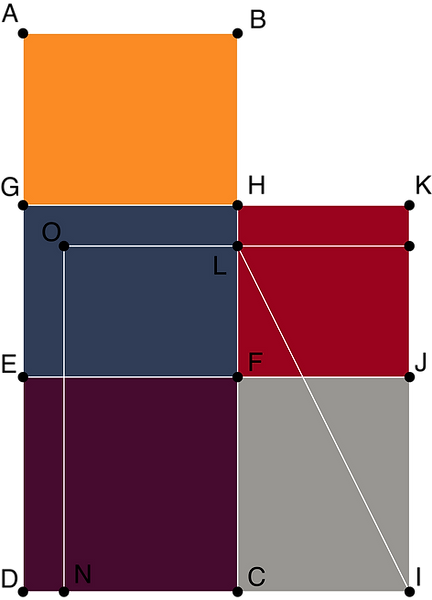top of page
##### Converting a Rectangle into a Sqaure

Baudhyana Shulbasutra describes a method of converting a square to a circle as:

दीर्घ चतुरस्त्रं समचतुरस्त्रं चिकीर्षंस्तिर्यङ्मानीं करणीं कृत्वा शेषं द्वेधा विभज्य पार्श्वयोरुपदध्यात्| खण्डमावापेन तत्संपूरयेत्| तस्य निर्हार उक्तः|| बौधायन शुल्बसुत्रम् १.५४||

“If you are desirous of converting an oblong into a square, cut off a square from the oblong by taking the tiryanmani of the oblong as its side; divide the remaining portions into two parts and adjust them along the two sides of the square. Complete the square by adding a smaller square in the corner. Deduct the added portion from the resultant figure”  Baudhayana Sulbasutra (1.54)

Construct a rectangle ABCD of length l and breadth b. Mark a point E on AD such that ED = CD. Similarly, mark a point F on CB such that CF = CD. Complete the square CDEF. Now bisect E at G and divide the rectangle ABFE into two equal parts. Move the square ABHG along CF. Now, taking DI as the side length, complete the square DIKG. Rotate IK about I such that it falls on HC at L. Make a point M on IK such that LM is parallel to HK. Taking MI as the new side, draw the square MINO. The square has an area equal to the rectangle ABCD.bottom of page